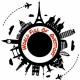Q15. Write a C++ program to take input in three subjects Physics, Chemistry and Math’s and calculate the total and percentage.

Solution:

#include<iostream.h> //standard input/output header file

#include<conio.h> //for clrscr()

int main()

{

int Phy,Chem,Maths,total,per;

clrscr(); //for clearing the screen

cout<<“\n Enter the marks of Physics: “;

cin>>Phy;

cout<<“\n Enter the marks of Chemistry: “;

cin>>Chem;

cout<<“\n Enter the marks of Math’s: “;

cin>>Maths;

total=Phy+Chem+Maths;

per=total/3;

cout<<“\n Total= “<<total;

cout<<“\n Percentage= “<<per<<“%”;

getch(); //holds the screen until any key is pressed

return 0; //makes main() finish

}

OUTPUT:

Enter the marks of Physics: 78

Enter the marks of Chemistry: 89

Enter the marks of Math’s: 90

Total= 257

Percentage= 85%#### WFQ

World is full of questions and we are trying to solve a few of them.

## 0 Comments

•### Madhvi Saxebna

Its very helpful